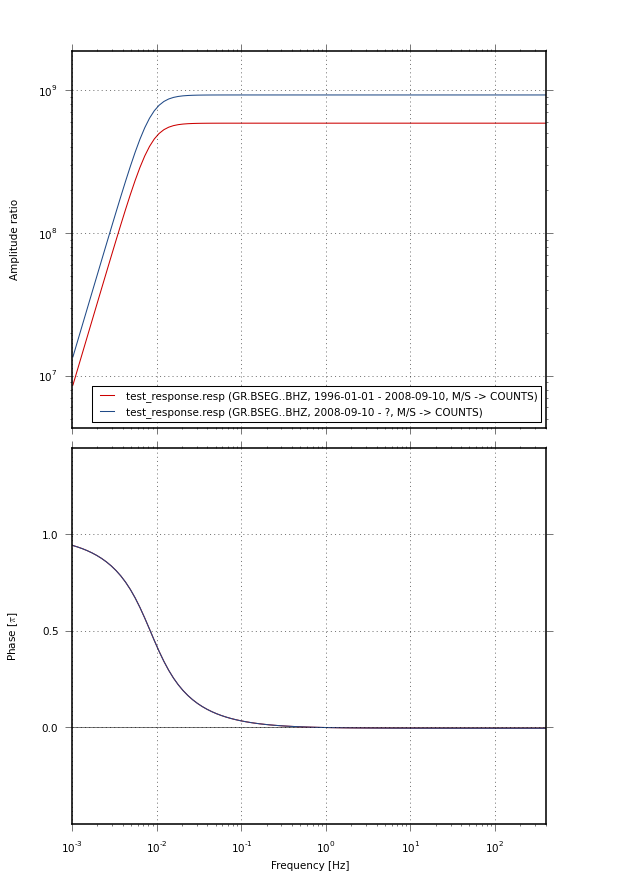# `plot.beachball`¶

exception `BeachballError`[source]
class `FixedPointOffsetTransform`(trans, dpi_scale_trans, fixed_point)[source]
`transform_non_affine`(values)[source]

Performs only the non-affine part of the transformation.

`transform(values)` is always equivalent to `transform_affine(transform_non_affine(values))`.

In non-affine transformations, this is generally equivalent to `transform(values)`. In affine transformations, this is always a no-op.

valuesarray

The input values as NumPy array of length `input_dims` or shape (N x `input_dims`).

valuesarray

The output values as NumPy array of length `input_dims` or shape (N x `output_dims`), depending on the input.

`plot_beachball_mpl`(mt, axes, beachball_type='deviatoric', position=0.0, 0.0, size=None, zorder=0, color_t='red', color_p='white', edgecolor='black', linewidth=2, alpha=1.0, arcres=181, decimation=1, projection='lambert', size_units='points', view='top')[source]

Plot beachball diagram to a Matplotlib plot

Parameters
• mt`pyrocko.moment_tensor.MomentTensor` object or an array or sequence which can be converted into an MT object

• beachball_type`'deviatoric'` (default), `'full'`, or `'dc'`

• position – position of the beachball in data coordinates

• size – diameter of the beachball either in points or in data coordinates, depending on the `size_units` setting

• zorder – (passed through to matplotlib drawing functions)

• color_t – color for compressional quadrants (default: `'red'`)

• color_p – color for extensive quadrants (default: `'white'`)

• edgecolor – color for lines (default: `'black'`)

• linewidth – linewidth in points (default: `2`)

• alpha – (passed through to matplotlib drawing functions)

• projection`'lambert'` (default), `'stereographic'`, or `'orthographic'`

• size_units`'points'` (default) or `'data'`, where the latter causes the beachball to be projected in the plots data coordinates (axes must have an aspect ratio of 1.0 or the beachball will be shown distorted when using this).

• view – View the beachball from `top`, `north`, `south`, `east` or `west`. Useful for to show beachballs in cross-sections. Default is `top`.

`plot_fuzzy_beachball_mpl_pixmap`(mts, axes, best_mt=None, beachball_type='deviatoric', position=0.0, 0.0, size=None, zorder=0, color_t='red', color_p='white', edgecolor='black', best_color='red', linewidth=2, alpha=1.0, projection='lambert', size_units='data', grid_resolution=200, method='imshow', view='top')[source]

Plot fuzzy beachball from a list of given MomentTensors

Parameters

See plot_beachball_mpl for other arguments

# `plot.cake_plot`¶

`path2colorint`(path)[source]

Calculate an integer representation deduced from path’s given name.

# `plot.hudson`¶

`project`(mt)[source]

Calculate Hudson’s (u, v) coordinates for a given moment tensor.

The moment tensor can be given as a `pyrocko.moment_tensor.MomentTensor` object, or by anything that can be converted to a 3x3 NumPy matrix, or as the six independent moment tensor entries as `(mnn, mee, mdd, mne, mnd, med)`.

`draw_axes`(axes, color='black', fontsize=12, linewidth=1.5)[source]

Plot axes and annotations of Hudson’s MT decomposition diagram.

# `plot.response`¶

This module contains functions to plot instrument response transfer functions in Bode plot style using Matplotlib.

Example

```from pyrocko.plot import response
from pyrocko.example import get_example_data

get_example_data('test_response.resp')

'test_response.resp', 'resp')

response.plot(
responses=resps, labels=labels, filename='test_response.png',
fmin=0.001, fmax=400., dpi=75.)
```Example response plot

`draw`(response, axes_amplitude=None, axes_phase=None, fmin=0.01, fmax=100.0, nf=100, normalize=False, style={}, label=None)[source]

Draw instrument response in Bode plot style to given Matplotlib axes

Parameters
`setup_axes`(axes_amplitude=None, axes_phase=None)[source]

Configure axes in Bode plot style.

`plot`(responses, filename=None, dpi=100, fmin=0.01, fmax=100.0, nf=100, normalize=False, fontsize=10.0, figsize=None, styles=None, labels=None)[source]

Draw instrument responses in Bode plot style.

Parameters